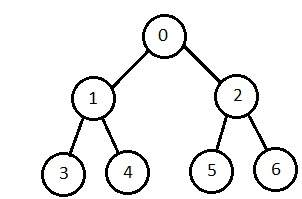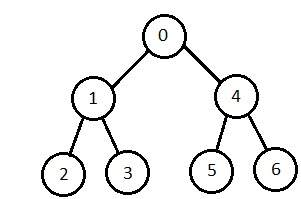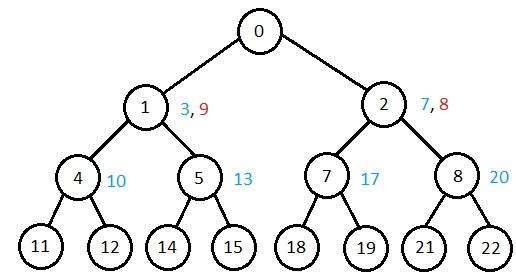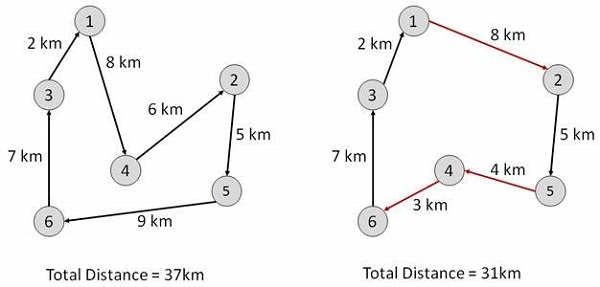# AI - Popular Search Algorithms

Searching is the universal technique of problem solving in AI. There are some single-player games such as tile games, Sudoku, crossword, etc. The search algorithms help you to search for a particular position in such games.

## Single Agent Pathfinding Problems

The games such as 3X3 eight-tile, 4X4 fifteen-tile, and 5X5 twenty four tile puzzles are single-agent-path-finding challenges. They consist of a matrix of tiles with a blank tile. The player is required to arrange the tiles by sliding a tile either vertically or horizontally into a blank space with the aim of accomplishing some objective.

The other examples of single agent pathfinding problems are Travelling Salesman Problem, Rubik’s Cube, and Theorem Proving.

## Search Terminology

• Problem Space − It is the environment in which the search takes place. (A set of states and set of operators to change those states)

• Problem Instance − It is Initial state + Goal state.

• Problem Space Graph − It represents problem state. States are shown by nodes and operators are shown by edges.

• Depth of a problem − Length of a shortest path or shortest sequence of operators from Initial State to goal state.

• Space Complexity − The maximum number of nodes that are stored in memory.

• Time Complexity − The maximum number of nodes that are created.

• Admissibility − A property of an algorithm to always find an optimal solution.

• Branching Factor − The average number of child nodes in the problem space graph.

• Depth − Length of the shortest path from initial state to goal state.

## Brute-Force Search Strategies

They are most simple, as they do not need any domain-specific knowledge. They work fine with small number of possible states.

Requirements −

• State description
• A set of valid operators
• Initial state
• Goal state description

It starts from the root node, explores the neighboring nodes first and moves towards the next level neighbors. It generates one tree at a time until the solution is found. It can be implemented using FIFO queue data structure. This method provides shortest path to the solution.

If branching factor (average number of child nodes for a given node) = b and depth = d, then number of nodes at level d = bd.

The total no of nodes created in worst case is b + b2 + b3 + … + bd.

Disadvantage − Since each level of nodes is saved for creating next one, it consumes a lot of memory space. Space requirement to store nodes is exponential.

Its complexity depends on the number of nodes. It can check duplicate nodes.### Depth-First Search

It is implemented in recursion with LIFO stack data structure. It creates the same set of nodes as Breadth-First method, only in the different order.

As the nodes on the single path are stored in each iteration from root to leaf node, the space requirement to store nodes is linear. With branching factor b and depth as m, the storage space is bm.

Disadvantage − This algorithm may not terminate and go on infinitely on one path. The solution to this issue is to choose a cut-off depth. If the ideal cut-off is d, and if chosen cut-off is lesser than d, then this algorithm may fail. If chosen cut-off is more than d, then execution time increases.

Its complexity depends on the number of paths. It cannot check duplicate nodes.### Bidirectional Search

It searches forward from initial state and backward from goal state till both meet to identify a common state.

The path from initial state is concatenated with the inverse path from the goal state. Each search is done only up to half of the total path.

### Uniform Cost Search

Sorting is done in increasing cost of the path to a node. It always expands the least cost node. It is identical to Breadth First search if each transition has the same cost.

It explores paths in the increasing order of cost.

Disadvantage − There can be multiple long paths with the cost ≤ C*. Uniform Cost search must explore them all.

### Iterative Deepening Depth-First Search

It performs depth-first search to level 1, starts over, executes a complete depth-first search to level 2, and continues in such way till the solution is found.

It never creates a node until all lower nodes are generated. It only saves a stack of nodes. The algorithm ends when it finds a solution at depth d. The number of nodes created at depth d is bd and at depth d-1 is bd-1.### Comparison of Various Algorithms Complexities

Let us see the performance of algorithms based on various criteria −

Criterion Breadth First Depth First Bidirectional Uniform Cost Interactive Deepening
Time bd bm bd/2 bd bd
Space bd bm bd/2 bd bd
Optimality Yes No Yes Yes Yes
Completeness Yes No Yes Yes Yes

## Informed (Heuristic) Search Strategies

To solve large problems with large number of possible states, problem-specific knowledge needs to be added to increase the efficiency of search algorithms.

### Heuristic Evaluation Functions

They calculate the cost of optimal path between two states. A heuristic function for sliding-tiles games is computed by counting number of moves that each tile makes from its goal state and adding these number of moves for all tiles.

### Pure Heuristic Search

It expands nodes in the order of their heuristic values. It creates two lists, a closed list for the already expanded nodes and an open list for the created but unexpanded nodes.

In each iteration, a node with a minimum heuristic value is expanded, all its child nodes are created and placed in the closed list. Then, the heuristic function is applied to the child nodes and they are placed in the open list according to their heuristic value. The shorter paths are saved and the longer ones are disposed.

### A * Search

It is best-known form of Best First search. It avoids expanding paths that are already expensive, but expands most promising paths first.

f(n) = g(n) + h(n), where

• g(n) the cost (so far) to reach the node
• h(n) estimated cost to get from the node to the goal
• f(n) estimated total cost of path through n to goal. It is implemented using priority queue by increasing f(n).

### Greedy Best First Search

It expands the node that is estimated to be closest to goal. It expands nodes based on f(n) = h(n). It is implemented using priority queue.

Disadvantage − It can get stuck in loops. It is not optimal.

## Local Search Algorithms

They start from a prospective solution and then move to a neighboring solution. They can return a valid solution even if it is interrupted at any time before they end.

### Hill-Climbing Search

It is an iterative algorithm that starts with an arbitrary solution to a problem and attempts to find a better solution by changing a single element of the solution incrementally. If the change produces a better solution, an incremental change is taken as a new solution. This process is repeated until there are no further improvements.

function Hill-Climbing (problem), returns a state that is a local maximum.

```inputs: problem, a problem
local variables: current, a node
neighbor, a node
current <-Make_Node(Initial-State[problem])
loop
do neighbor <- a highest_valued successor of current
if Value[neighbor] ≤ Value[current] then
return State[current]
current <- neighbor

end
```

Disadvantage − This algorithm is neither complete, nor optimal.

### Local Beam Search

In this algorithm, it holds k number of states at any given time. At the start, these states are generated randomly. The successors of these k states are computed with the help of objective function. If any of these successors is the maximum value of the objective function, then the algorithm stops.

Otherwise the (initial k states and k number of successors of the states = 2k) states are placed in a pool. The pool is then sorted numerically. The highest k states are selected as new initial states. This process continues until a maximum value is reached.

function BeamSearch( problem, k), returns a solution state.

```start with k randomly generated states
loop
generate all successors of all k states
if any of the states = solution, then return the state
else select the k best successors
end
```

### Simulated Annealing

Annealing is the process of heating and cooling a metal to change its internal structure for modifying its physical properties. When the metal cools, its new structure is seized, and the metal retains its newly obtained properties. In simulated annealing process, the temperature is kept variable.

We initially set the temperature high and then allow it to ‘cool' slowly as the algorithm proceeds. When the temperature is high, the algorithm is allowed to accept worse solutions with high frequency.

Start

• Initialize k = 0; L = integer number of variables;
• From i → j, search the performance difference Δ.
• If Δ <= 0 then accept else if exp(-Δ/T(k)) > random(0,1) then accept;
• Repeat steps 1 and 2 for L(k) steps.
• k = k + 1;

Repeat steps 1 through 4 till the criteria is met.

End

### Travelling Salesman Problem

In this algorithm, the objective is to find a low-cost tour that starts from a city, visits all cities en-route exactly once and ends at the same starting city.

```Start
Find out all (n -1)! Possible solutions, where n is the total number of cities.
Determine the minimum cost by finding out the cost of each of these (n -1)! solutions.
Finally, keep the one with the minimum cost.
end
```